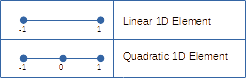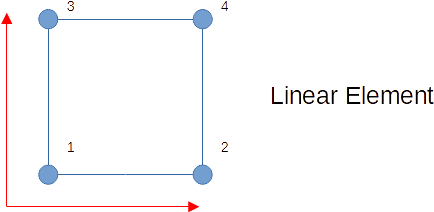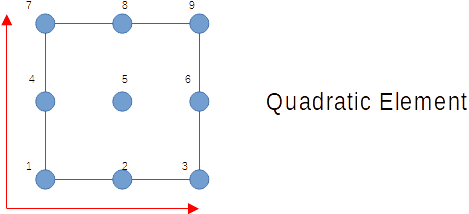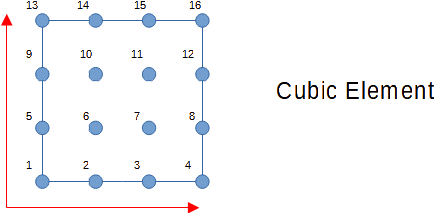# Visualizing 1D & 2D FEM Basis Functions in Julia

The Finite Element Method (FEM) is a mathematical tool using for solving differential equations. It has been popularly used (also, initially developed) for solving elastostatics problems which is to find the displacement $u$ such that, $$\sigma_{ij,j} + f_i = 0 \text{ in the domain }\Omega$$ To solve this, the domain $\Omega$ is split into finite sub domains $\Omega_e$ in which the solution $u$ is (usually) approximated by a polynomial of certain order which is governed by the regularity requirements of the weak form of above PDE.

The division into finite elements $\Omega_e$ is usually done as

• Lines for 1D problem (obviously)
• Quadrilaterals in 2D problems
• Hexahedrons in 3D problems

These finite elements are then mapped to a “Natural co-ordinate” system where they accordingly become

• Lines with $\xi_1 \in (-1,1)$
• Square with $\xi_1, \xi_2 \in (-1,1)$
• Cubes with $\xi_1, \xi_2, \xi_3 \in (-1,1)$

where $\xi_1, \xi_2, \xi_3$ are the $x,y,z$ co-ordinates in this bi-unit domain (called so since these values here range from $-1$ to $1$).

While the FEM is solved for discrete values of $u$, the solution is presented as a continuous function over the domain as well as within the elements. This equation of the solution is obtained by scaling the Lagrangian polynomials in this space by the nodal values of $u$ (it is these nodal values that FEM finally solves for). These Lagrangian polynomials are (usually) the basis functions of the finite elements.

## 1-D Elements

SeeThe above diagram shows the nodes for 1D elements. There are two main points to be noted:

• There are polynomials for each node of an element. And these polynomials are chosen such a way that these polynomials have value $1$ at that node and $0$ at all other nodes.
• Order of the polynomial $=\text{Number of nodes}-1$

The final solution is the sum of all these polynomials which can be given as $$u(\xi) = \sum_{A=1}^{N_{\text{nodes}}}N_A(\xi)\bf u_A$$ where,

• $N_A$ is the basis function at node A.
• $\bf u_A$ is nodal degree of freedom (value of displacement).
• $N_\text{nodes}$ is the total number of nodes per element.

The general Lagrangian basis function $N_A$ is given as, $$N_A(\xi) = \prod_{B=1 \\ B\not=A}^{N_\text{nodes}}\frac {\xi - \xi_B}{\xi_A - \xi_B}$$ where $B$ iterates through all the nodes except A. It can be observed here that the value of basis function is always zero at all the nodes (except A).

  1 2 3 4 5 6 7 8 9 10 11 12  # The generic Lagrangian basis function is function N(ξ, order, node) ξ_B = range(-1, 1, length=order+1) |> collect ξ_A = ξ_B[node] N_A = 1 for i in range(1, order+1) if i ≠ node N_A *= (ξ - ξ_B[i])/(ξ_A - ξ_B[i]) end end N_A end 

Note that, we won’t be using the generic function for the ease of comparison of various orders. Now that we are moving into plotting of basis functions, let me initialize the prerequisites in Julia.

 1 2 3 4  using Plots plotly() ξ₁ = ξ₂ = range(-1, 1, step=0.01) |> collect 

### 1D Linear Element

For linear elements, substituting the nodal $\xi$ values in the above basis function, we get the shape functions as follows,

$$N_1^1(\xi) = \frac {1-\xi}{2} \\ N_2^1(\xi) = \frac {1+\xi}{2}$$

The superscript 1 denotes that it is a linear element. The same basis functions can be obtained by calling the above function as N(ξ, 1, node) for each of the respective node. The corresponding plots are,

 1 2 3 4 5 6 7 8  N₁1(ξ) = (1-ξ)/2 N₂1(ξ) = (1+ξ)/2 plt1 = plot(N₁1, -1, 1, title="N₁") plt2 = plot(N₂1, -1, 1, title = "N₂") plot(plt1, plt2, legend=false, xlabel="ξ₁", ylabel="N") 

### 1D Quadratic Element

The quadratic shape function is given as,

\begin{aligned} N_1^2(\xi) &= \frac {\xi^2-\xi}{2} \\ N_2^2(\xi) &= \frac {\xi^2}{2} \\ N_2^2(\xi) &= \frac {\xi^2+\xi}{2} \end{aligned}
Again, the superscript $2$ denotes that its order is 2.
  1 2 3 4 5 6 7 8 9 10  N₁2(ξ) = (ξ-1)ξ/2 N₂2(ξ) = 1-ξ^2 N₃2(ξ) = (ξ+1)ξ/2 plt1 = plot(N₁2, ξ₁, title="N₁") plt2 = plot(N₂2, ξ₁, title = "N₂") plt3 = plot(N₃2, ξ₁, title = "N₃") plot(plt1, plt2, plt3, legend=false, xlabel="ξ₁", ylabel="N") 

### 1D Cubic Element

The cubic shape function is,

\begin{aligned} N_1^3(\xi) &= \frac{-1}{16}(9\xi^3 - 9\xi^2 - \xi + 1) \\ N_2^3(\xi) &= \frac{1}{16}(27\xi^3 -9\xi^2 -27\xi + 9) \\ N_2^3(\xi) &= \frac{-1}{16}(27\xi^3 + 9\xi^2 - 27\xi - 9) \\ N_4^3(\xi) &= \frac{1}{16}(9\xi^3 + 9\xi^2 - \xi - 1) \end{aligned}
  1 2 3 4 5 6 7 8 9 10 11 12  N₁3(ξ) = -(9ξ^3 - 9ξ^2 - ξ + 1)/16 N₂3(ξ) = (27ξ^3 -9ξ^2 -27ξ + 9)/16 N₃3(ξ) = -(27ξ^3 + 9ξ^2 -27ξ - 9)/16 N₄3(ξ) = (9ξ^3 + 9ξ^2 - ξ - 1)/16 plt1 = plot(N₁3, ξ₁, title="N₁") plt2 = plot(N₂3, ξ₁, title = "N₂") plt3 = plot(N₃3, ξ₁, title = "N₃") plt4 = plot(N₄3, ξ₁, title = "N₄") plot(plt1, plt2, plt3, plt4, legend=false, xlabel="ξ₁", ylabel="N") 

## 2D Elements

For the case of 2D elements, we will consider the quadratic elements which can be obtained as a tensor product of the above 1D basis functions. This can be represented as,

\begin{aligned} \bf N &= N^{\xi_1} \otimes N^{\xi_2}\\ \bf N_{ij} &= N_i N_j \end{aligned}
Generally, an element has the same order of the basis functions along both $\xi_1$ and $\xi_2$ directions though it need not be the case.

Thus, for a quadilateral element, the basis function in the natural co-ordinates (where it is transformed to square elements) over each node can be obtained by tensor product as,

\begin{aligned} \mathbf{N} &=\left[\begin{array}{c} N_{1} \\ N_{2} \\ \vdots \\ N_{n} \end{array}\right] \otimes \left[\begin{array}{c} N_{1} \\ N_{2} \\ \vdots \\ N_{n} \end{array}\right] \\ &= \left[\begin{array}{cccc} N_{1} N_{1} & N_{1} N_{2} & \cdots & N_{1} N_{n} \\ N_{2} N_{1} & N_{2} N_{2} & \cdots & N_{2} N_{n} \\ \vdots & \vdots & \ddots & \vdots \\ N_{n} N_{1} & N_{n} N_{2} & \cdots & N_{n} N_{n} \end{array}\right] \end{aligned}

### 2D Linear ElementsFor the linear elements, following the previous notations we can obtain the shape function as,

$$\bf{N} =\left[\begin{array}{c} N_{1} \\ N_{2} \end{array}\right] \otimes \left[\begin{array}{c} N_{1} \\ N_{2} \\ \end{array}\right] = \left[\begin{array}{cc} N_{1} N_{1} & N_{1} N_{2} \\ N_{2} N_{1} & N_{2} N_{2} \end{array}\right]$$
In the same spirits, the julia code can be created as the outer outer of the 1D linear element vector as
 1 2  N1(ξ) = [N₁1(ξ), N₂1(ξ)] N2(ξ1, ξ2) = N1(ξ1)*N1(ξ2)' 

But, for the readability, we will follow the elementwise multiplication as instead of the above seen code.

 1 2 3 4 5 6 7 8 9  N₁₁1(ξ1, ξ2) = N₁1(ξ1)*N₁1(ξ2) N₁₂1(ξ1, ξ2) = N₁1(ξ1)*N₂1(ξ2) N₂₁1(ξ1, ξ2) = N₂1(ξ1)*N₁1(ξ2) N₂₂1(ξ1, ξ2) = N₂1(ξ1)*N₂1(ξ2) s11=surface(ξ₁, ξ₂, N₁₁1, title="N₁₁") s21=surface(ξ₁, ξ₂, N₁₂1, title="N₁₂") s31=surface(ξ₁, ξ₂, N₂₁1, title="N₂₁") s41=surface(ξ₁, ξ₂, N₂₂1, title="N₂₂") plot(s11,s21,s31,s41) 

Here is the plot for the $N_{11}$. The $z$ axis represents the nodal values of the shape function while the $xy$ plane represents the vector field of $\xi_1$ and $\xi_2$.

One interesting point to observe here is that, though the function is linear, the plot is curved along the diagonal. That is because the shape function is actually a bilinear function of $\xi_1$ and $\xi_2$. Here is the plot for each shape functions.

### 2D Quadratic ElementsThe quadratic elements are obtained through the tensor product as,

$$\bf{N} =\left[\begin{array}{c} N_{1} \\ N_{2} \\ N_{3} \end{array}\right] \otimes \left[\begin{array}{c} N_{1} \\ N_{2} \\ N_{3} \end{array}\right] = \left[\begin{array}{ccc} N_{1} N_{1} & N_{1} N_{2} & N_{1} N_{3}\\ N_{2} N_{1} & N_{2} N_{2} & N_{2} N_{3}\\ N_{3} N_{1} & N_{3} N_{2} & N_{3} N_{3} \end{array}\right]$$
  1 2 3 4 5 6 7 8 9 10 11 12 13 14 15 16 17 18 19  N₁₁2(ξ1, ξ2) = N₁2(ξ1)*N₁2(ξ2) N₁₂2(ξ1, ξ2) = N₁2(ξ1)*N₂2(ξ2) N₁₃2(ξ1, ξ2) = N₁2(ξ1)*N₃2(ξ2) N₂₁2(ξ1, ξ2) = N₂2(ξ1)*N₁2(ξ2) N₂₂2(ξ1, ξ2) = N₂2(ξ1)*N₂2(ξ2) N₂₃2(ξ1, ξ2) = N₂2(ξ1)*N₃2(ξ2) N₃₁2(ξ1, ξ2) = N₃2(ξ1)*N₁2(ξ2) N₃₂2(ξ1, ξ2) = N₃2(ξ1)*N₂2(ξ2) N₃₃2(ξ1, ξ2) = N₃2(ξ1)*N₃2(ξ2) s12=surface(ξ₁, ξ₂, N₁₁2, title="N₁₁") s22=surface(ξ₁, ξ₂, N₁₂2, title="N₁₂") s32=surface(ξ₁, ξ₂, N₁₃2, title="N₁₃") s42=surface(ξ₁, ξ₂, N₂₁2, title="N₂₁") s52=surface(ξ₁, ξ₂, N₂₂2, title="N₂₂") s62=surface(ξ₁, ξ₂, N₂₃2, title="N₂₃") s72=surface(ξ₁, ξ₂, N₃₁2, title="N₃₁") s82=surface(ξ₁, ξ₂, N₃₂2, title="N₃₂") s92=surface(ξ₁, ξ₂, N₃₃2, title="N₃₃") plot(s12,s22, s32, s42, s52, s62, s72, s82, s92) 

### 2D Cubic Elements1 2 3 4 5 6 7 8 9 10 11 12 13 14 15 16 17 18 19 20 21 22 23 24 25 26 27 28 29 30 31 32 33  N₁₁3(ξ1, ξ2) = N₁3(ξ1)*N₁3(ξ2) N₁₂3(ξ1, ξ2) = N₁3(ξ1)*N₂3(ξ2) N₁₃3(ξ1, ξ2) = N₁3(ξ1)*N₃3(ξ2) N₁₄3(ξ1, ξ2) = N₁3(ξ1)*N₄3(ξ2) N₂₁3(ξ1, ξ2) = N₂3(ξ1)*N₁3(ξ2) N₂₂3(ξ1, ξ2) = N₂3(ξ1)*N₂3(ξ2) N₂₃3(ξ1, ξ2) = N₂3(ξ1)*N₃3(ξ2) N₂₄3(ξ1, ξ2) = N₂3(ξ1)*N₄3(ξ2) N₃₁3(ξ1, ξ2) = N₃3(ξ1)*N₁3(ξ2) N₃₂3(ξ1, ξ2) = N₃3(ξ1)*N₂3(ξ2) N₃₃3(ξ1, ξ2) = N₃3(ξ1)*N₃3(ξ2) N₃₄3(ξ1, ξ2) = N₃3(ξ1)*N₄3(ξ2) N₄₁3(ξ1, ξ2) = N₄3(ξ1)*N₁3(ξ2) N₄₂3(ξ1, ξ2) = N₄3(ξ1)*N₂3(ξ2) N₄₃3(ξ1, ξ2) = N₄3(ξ1)*N₃3(ξ2) N₄₄3(ξ1, ξ2) = N₄3(ξ1)*N₄3(ξ2) s013=surface(ξ₁, ξ₂, N₁₁3, title="N₁₁") s023=surface(ξ₁, ξ₂, N₁₂3, title="N₁₂") s033=surface(ξ₁, ξ₂, N₁₃3, title="N₁₃") s043=surface(ξ₁, ξ₂, N₁₄3, title="N₁₄") s053=surface(ξ₁, ξ₂, N₂₁3, title="N₂₁") s063=surface(ξ₁, ξ₂, N₂₂3, title="N₂₂") s073=surface(ξ₁, ξ₂, N₂₃3, title="N₂₃") s083=surface(ξ₁, ξ₂, N₂₄3, title="N₂₄") s093=surface(ξ₁, ξ₂, N₃₁3, title="N₃₁") s103=surface(ξ₁, ξ₂, N₃₂3, title="N₃₂") s113=surface(ξ₁, ξ₂, N₃₃3, title="N₃₃") s123=surface(ξ₁, ξ₂, N₃₄3, title="N₃₄") s133=surface(ξ₁, ξ₂, N₄₁3, title="N₄₁") s143=surface(ξ₁, ξ₂, N₄₂3, title="N₄₂") s153=surface(ξ₁, ξ₂, N₄₃3, title="N₄₃") s163=surface(ξ₁, ξ₂, N₄₄3, title="N₄₄") plot(s133,s143,s153,s163, s093,s103,s113,s123, s053,s063,s073,s083, s013,s023,s033,s043, colorbar=false, size=(600, 800)) 

Again, the cubic 2D element is the tensor product of the 1D shape functions,

$$\bf{N} =\left[\begin{array}{c} N_{1} \\ N_{2} \\ N_{3} \\ N_{4} \end{array}\right] \otimes \left[\begin{array}{c} N_{1} \\ N_{2} \\ N_{3} \\ N_{4} \end{array}\right] = \left[\begin{array}{cccc} N_{1} N_{1} & N_{1} N_{2} & N_{1} N_{3} & N_{1} N_{4}\\ N_{2} N_{1} & N_{2} N_{2} & N_{2} N_{3} & N_{2} N_{4}\\ N_{3} N_{1} & N_{3} N_{2} & N_{3} N_{3} & N_{3} N_{4}\\ N_{4} N_{1} & N_{4} N_{2} & N_{4} N_{3} & N_{4} N_{4}\\ \end{array}\right]$$

Thus a general representation of the Lagrangian basis functions have been achieved for the 1D and 2D elements. I do wish to implement the same for the 3D elements but I seem to be running out of spaces! (Actually, it is possible and will implement and update with link soon). This had been a very good learning experience for me and am looking forward to dwelving more into this realm soon!